### Data handling - Worksheets

CBSE Worksheet 01

Ch-9 Data handling
1. Following frequency distribution table shows marks (out of 50) obtained in English by 45 students of class VI. Which two classes have the same frequency?
 Class Interval Frequency 0 - 10 1 10 - 20 6 20 - 30 20 30 - 40 12 40 - 50 6 Total 45
1. 10 - 20 and 40 - 50
2. None of these
3. 10 - 20 and 20 - 30
4. 20 - 30 and 40 - 50
2. The pictograph shows the numbers of goals scored by four soccer teams in a season. How many goals did Kickers score?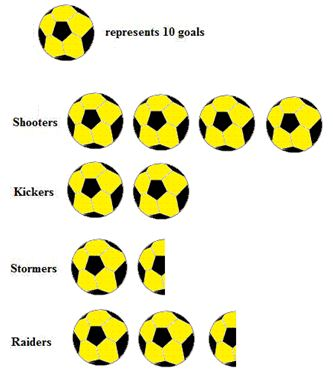1. 20
2. None of these
3. 10
4. 15
3. The following pictograph shows the number of absentees in a class of 50 students during the previous week. On which day were the maximum number of students absent?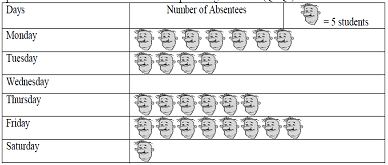1. Saturday
2. Friday
3. Thursday
4. Wednesday
4. A _______ is a collection of numbers gathered to give some information.
1. Tally mark
2. Data
3. None of these
4. Frequency
5. In a village six fruit merchants sold the following number of fruit baskets in a particular season. How many fruit baskets were sold by Rahim?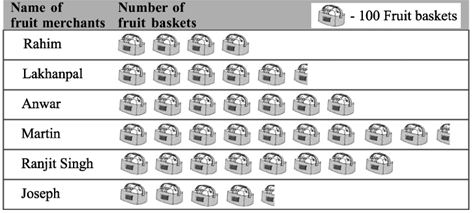1. 700
2. 400
3. 500
4. 650
6. This chart shows the number of people with birthdays in each three months of the year. How many people have a birthday before July?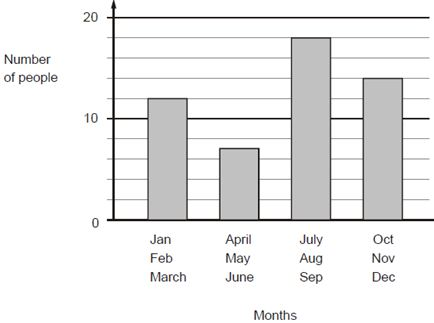1. None of these
2. 7
3. 12
4. 19
7. Match the following:-
Column AColumn B
1.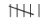(a) 8
2.(b) 6
3. |||(c) 5
4.(d) 3
8. Fill up the following:
1. Representation of data with the help of tally marks is called _________.
2. In a bar graph width of rectangle is always _________.
3. The tally markrepresents ________.
4. In a bar graph, _______ can be drawn horizontally and vertically.
9. State true or false:
1. A bar graph represents data in the form of pictures, object or parts of objects.
2. Data is a collection of numerical figures giving required information.
3. In a bar graph width of rectangle is always equal.
4. The tally markrepresents 5.
10. A collection of numbers gathered to give some information is called?
11. For a math assignment a group of students had to draw their favorite shapes.
The following pictures represent their choices. Each picture stands for 25 shapes.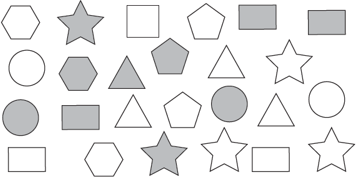12. The colors of fridges preferred by people living in a locality are shown by the following pictograph
Which colour most liked by the people?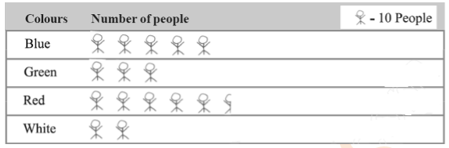13. In a village six fruit merchants sold the following number of fruit baskets in a particular season: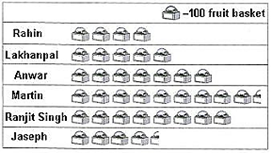Observe this pictograph and answer the following questions:
1. Which merchant sold the maximum number of baskets?
3. The merchants who have sold 600 or more number of baskets are planning to buy a godown for the next season. Can you name them?
14. A survey of 120 school students was done to find which activity they prefer to do in their free time :
Preferred activityNumber of Students
Plying45
Watching T.V.20
Listening music10
Painting15
Draw a bar graph to illustrate the above data taking scale of 1 unit length = 5 students.Which activity is preferred by most of the students other than playing ?
15. The bar graph shows the number of toys produced by a factory during a certain week: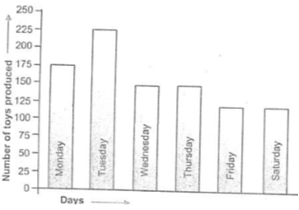1. On which day the maximum number of toys were produced?
2. On which day equal number of toys were produced?
3. What is the total number of toys produced during the week?
4. In which day minimum number of toys were produced?

CBSE Worksheet 01
Ch-9 Data handling

1. 10 - 20 and 40 - 50
Explanation: Both have 6 frequency
1. 20
Explanation: 2$×$10=20
1. Friday
Explanation: As friday has number of absentees 8 $×$ 5 = 40. highest number in the week.
1. Data
Explanation: collection of data =information
facts and statistics collected together for reference or analysis is called data
1. 400
Explanation: 4$×$100 = 400
1. 19
Explanation: 12+7 = 19
1. $\to$ (b)
2. $\to$ (a)
3. $\to$ (d)
4. $\to$ (c)
1. frequency distribution table
2. equal
3. 5
4. bar
1. False; A pictograph represents data in the form of pictures, object or parts of objects.
2. True
3. True
4. True
1. Data is collection of numbers gathered to give some information.
2. Total pictures = 25
Each picture stands for 25 shapes.
So, Total shapes students drew altogether = 25 $×$ 25 = 625 shapes
3. Number of people liked Red colour = 5 $×$ 10 + 5 = 55
Number of people liked White colour = 2 $×$ 10 = 20
Number of people liked Green colour = 3 $×$ 10 = 30
Number of people liked Blue colour = 5 $×$ 10 = 20
Hence, Red colour most liked by the people.
1. martin sold the maximum number of baskets.
2. $×$ 100 = 700 fruit baskets were sold by Answer.
3. Answer, Martin and Ranjit Singh are planning to buy a godown for the next season.
1. Draw two perpendicular lines - one vertical and one horizontal.
2. Along horizontal line mark the "Preferred activity" and along vertical line mark the "No. of students".
3. Take bars of same width keeping uniform gap between them.
4. Take scale of 1 unit length = 5 students along the vertical line and then mark the corresponding values.
5. Calculate the heights of the bars for various activities preferred as shown below :
 Playing 45 ÷ 5 = 9 units Reading story books 30 ÷ 5 = 6 units Watching T.V. 20 ÷ 5 = 4 units Listening music 10 ÷ 5 = 2 units Painting 15 ÷ 5 = 3 units
6. Now draw various bars.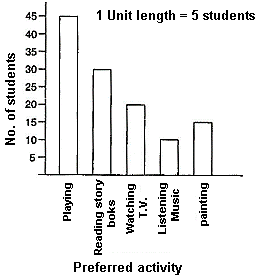The activity "Reading story books" is preferred by most of the students other than playing.
1. The maximum number of toys were produced on Tuesday.
2. Wednesday and Thursday, Friday and Saturday have equal number of toys were produced.
3. Total number of toys produced in the week = 175 + 225 + 150 + 150 + 125 + 125 = 900
4. Minimum number of toys were produced on Friday and Saturday.